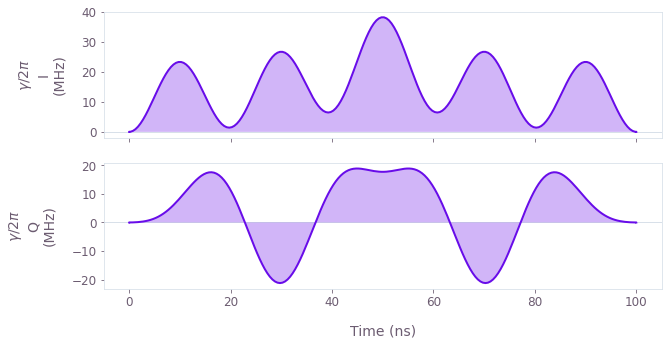•Boulder Opal
• User guides
• Optimize controls using a Hann series basis

# How to optimize controls using a Hann series basis

Create optimized controls using Hann series basis functions

Boulder Opal exposes a highly-flexible optimization engine for general-purpose gradient-based optimization. The controls can be described in terms of optimizable linear combinations from a set of built in (or user-defined) basis functions, which can greatly reduce the dimensionality of the optimization search space. In this notebook we will use Hann window functions, although the same technique has also seen success with other bases, for example Slepian functions. You can also read the related user guides showing how to find optimal controls using a Fourier basis or an arbitrary (user-defined) basis.

## Summary workflow

### 1. Define basis function for signal composition in the graph

The Boulder Opal signal library provides convenience graph operations to create piecewise-constant (PWC) and sampleable (STF) optimizable signals in a Hann series basis. To create the PWC Hann series use graph.signals.hann_series_pwc, which requires the duration, segment_count and set of coefficients (which may be optimizable tensors) to be specified. To create the STF Hann series use graph.signals.hann_series_stf, which requires the start_time, end_time and set of coefficients (which may be optimizable tensors) to be specified.

### 2. Execute graph-based optimization

With the graph object created, an optimization can be run using the qctrl.functions.calculate_optimization function. The cost, the outputs, and the graph must be provided. The function returns the results of the optimization.

The Boulder Opal Toolkits are currently in beta phase of development. Breaking changes may be introduced.

## Example: Robust optimization on a qutrit using Hann window functions

In this example, we perform optimization for a robust single qubit Hadamard gate (in the Hann window basis) of a qutrit system while minimizing leakage out of the computational subspace. The system is described by the following Hamiltonian:

$$H(t) = \frac{\chi}{2} (a^\dagger)^2 a^2 + (1+\beta(t)) \left(\gamma(t) a + \gamma^*(t) a^\dagger \right),$$

where $\chi$ is the anharmonicity, $\gamma(t)$ is a complex time-dependent signal, $\beta(t)$ is a small, slowly-varying stochastic amplitude noise process, and $a = |0 \rangle \langle 1 | + \sqrt{2} |1 \rangle \langle 2 |$.

We parametrize the signal $\gamma(t) = \gamma_I(t) + i \gamma_Q(t)$ in terms of Hann window functions:

$$\gamma_{I(Q)}(t) = \sum_{n=1}^N{\frac{c^{I(Q)}_n}{2} \left[1-\cos\left(\frac{2\pi nt}{\tau_g}\right) \right]},$$

where $c^{I(Q)}_n$ are the different real-valued coefficients describing the parametrization and $\tau_g$ is the gate duration. This is a good choice for implementation in bandwidth-limited hardware as it is composed of smooth functions that go to zero at the edges.

import numpy as np

from qctrlvisualizer import plot_controls
from qctrl import Qctrl

# Start a Boulder Opal session.
qctrl = Qctrl()
# Define target and projector matrices.
hadamard = np.array([[1.0, 1.0, 0], [1.0, -1.0, 0], [0, 0, np.sqrt(2)]]) / np.sqrt(2)
qubit_projector = np.diag([1.0, 0.0, 0.0])

# Define physical constraints.
chi = -2 * np.pi * 300.0 * 1e6  # Hz
gamma_max = 2 * np.pi * 50e6  # Hz
segment_count = 200
duration = 100e-9  # s
sample_times = np.linspace(0, duration, segment_count)

optimizable_frequency_count = 5

# Create graph object.
graph = qctrl.create_graph()

# Define standard matrices.
a = graph.annihilation_operator(3)

# Define the coefficients of the Hann functions for optimization.
hann_coefficients_i = graph.optimization_variable(
optimizable_frequency_count, lower_bound=-1, upper_bound=1
)
hann_coefficients_q = graph.optimization_variable(
optimizable_frequency_count, lower_bound=-1, upper_bound=1
)
hann_coefficients = gamma_max * (hann_coefficients_i + 1j * hann_coefficients_q)

# Create gamma(t) signal in Hann function basis.
gamma = graph.signals.hann_series_pwc(
duration=duration,
segment_count=segment_count,
coefficients=hann_coefficients,
name=r"$\gamma$",
)

# Create anharmonicity term.

# Create drive term.
drive = graph.hermitian_part(2 * gamma * a)

# Create target operator in qubit subspace.
target_operator = graph.target(
)

# Create infidelity.
infidelity = graph.infidelity_pwc(
hamiltonian=anharmonicity + drive,
target=target_operator,
noise_operators=[drive],
name="infidelity",
)

# Run the optimization and retrieve results.
optimization_result = qctrl.functions.calculate_optimization(
cost_node_name="infidelity",
output_node_names=[r"$\gamma$"],
graph=graph,
optimization_count=4,
)

print(f"\nOptimized cost:\t{optimization_result.cost:.3e}")

plot_controls(optimization_result.output, smooth=True, polar=False)
Your task calculate_optimization (action_id="1599304") is currently in a queue waiting to be processed.

Optimized cost:	2.329e-06This notebook was run using the following package versions. It should also be compatible with newer versions of the Q-CTRL Python package.

PackageVersion
Python3.10.8
matplotlib3.6.3
numpy1.24.1
scipy1.10.0
qctrl20.1.1
qctrl-commons17.7.0
boulder-opal-toolkits2.0.0-beta.3
qctrl-visualizer4.4.0